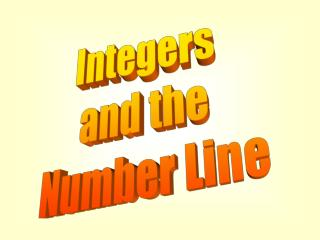DownloadDownload PresentationIntegers and the Number Line

Integers and the Number Line

Télécharger la présentationIntegers and the Number Line

- - - - - - - - - - - - - - - - - - - - - - - - - - - E N D - - - - - - - - - - - - - - - - - - - - - - - - - - -
Presentation Transcript

1. Integers and the Number Line

2. Number Lines -5 0 5 -10 10 A number line is a line with marks on it that are placed at equal distances apart. One mark on the number line is usually labeled zero and then each successive mark to the left or to the right of the zero represents a particular unit such as 1 or ½. On the number line above, each small mark represents ½ unit and the larger marks represent 1 unit.

3. Number Lines -5 0 5 -10 10 • Number lines can be used to represent: • Whole numbers – The set {0, 1, 2, 3, …}. • Positive numbers – any number that is greater than zero. • Negative numbers – any number that is less than zero. • Integers – The set of numbers represented as • {…, -3, -2, -1, 0, 1, 2, 3, …} The arrows at the ends of the number line show that the number line continues in both directions without ending.

4. Graphing Numbers -5 0 5 -10 10 A number can be graphed on a number line by placing a point at the appropriate position on the number line. • Example • {4} (blue point) • {integers between –10 and –5} (purple)

5. Graphing Numbers -5 0 5 -10 10 Name the set of numbers that is graphed. {-8, -4, 1, 5, 8}

6. Moving On A Number Line -5 0 5 -10 10 Movement to the right on the number line is in the positive direction (increasing). Movement to the left on the number line is in the negative direction (decreasing). Make the following moves on the number line. Start at 5 and move left 7. -2 Where did you stop? How can we represent this mathematically? 5 + (-7) = -2

7. You Try It Graph the set of numbers on a number line. Write two inequalities comparing the two numbers. 1. -2, 7 2. -9, -4 3. 3, 8 Find each sum using a number line. • 3 + 7 5. -1 + (-7) • -4 + 12 7. -9 + 5 • 8. -6 + 6

8. -5 -5 -5 0 0 0 5 5 5 -10 -10 -10 10 10 10 Problems 1-3

9. Problem 4 -5 0 5 -10 10 3 + 7 Start at 3 and move 7 places to the right. 3 + 7 = 10

10. Problem 5 -5 0 5 -10 10 -1 +(-7) Start at -1 and move 7 places to the left. -1 + (-7) = -8

11. Problem 6 -5 0 5 -10 10 -4 + 12 Start at -4 and move 12 places to the right. -4 + 12 = 8

12. Problem 7 -5 0 5 -10 10 -9 + 5 Start at -9 and move 5 places to the right. -9 + 5 = -4

13. Problem 8 -5 0 5 -10 10 -6 + 6 Start at -6 and move 6 places to the right. -6 + 6 = 0